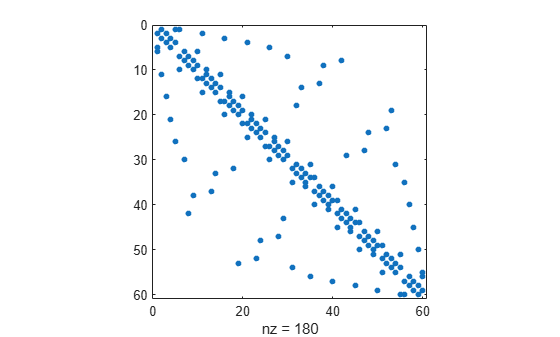# sprandn

Sparse normally distributed random matrix

## Syntax

``R = sprandn(S)``
``R = sprandn(m,n,density)``
``R = sprandn(m,n,density,rc)``

## Description

example

````R = sprandn(S)` creates a sparse matrix that has the same sparsity pattern as the matrix `S`, but with normally distributed random entries with mean `0` and variance `1`.```

example

````R = sprandn(m,n,density)` creates a random `m`-by-`n` sparse matrix with approximately `density*m*n` normally distributed nonzero entries for `density` in the interval [0,1].```

example

````R = sprandn(m,n,density,rc)` creates a matrix that also has reciprocal condition number approximately equal to `rc`. The matrix `R` is constructed from a sum of matrices of rank one. ```

## Examples

collapse all

Create the `60`-by-`60` sparse adjacency matrix of the connectivity graph of the Buckminster Fuller geodesic dome. Plot the sparsity pattern of the matrix `S`.

```S = bucky; spy(S)```Create another sparse matrix `R` that has the same sparsity pattern as the matrix `S`, but with normally distributed random entries. Plot the sparsity pattern of `R`.

```R = sprandn(S); spy(R)```Create a random `500`-by-`1000` sparse matrix with density `0.1`. The matrix has approximately `0.1*500*1000 = 50000` normally distributed nonzero entries.

`R = sprandn(500,1000,0.1);`

Show the exact number of nonzero elements in the matrix `R`.

`n = nnz(R)`
```n = 47663 ```

Create a random `50`-by-`100` sparse matrix with approximately `0.2*50*100 = 1000` normally distributed nonzero entries. Specify the reciprocal condition number of the matrix to be `0.25`.

`R = sprandn(50,100,0.2,0.25);`

Show that the condition number of the matrix `R` is equal to `1/0.25 = 4`.

`cond(full(R))`
```ans = 4.0000 ```

## Input Arguments

collapse all

Input matrix, specified as a full or sparse matrix.

Data Types: `single` | `double` | `int8` | `int16` | `int32` | `int64` | `uint8` | `uint16` | `uint32` | `uint64` | `logical`
Complex Number Support: Yes

Number of matrix rows, specified as a nonnegative integer.

Number of matrix columns, specified as a nonnegative integer.

Density of nonzero elements, specified as a scalar. `density` must be in the interval [0,1].

Data Types: `double`

Reciprocal condition number, specified as a scalar or vector. `rc` must be in the interval [0,1].

If `rc` is a vector of length `lr`, where `lr <= min(m,n)`, then ```R = sprandn(m,n,density,rc)``` has `rc` as its first `lr` singular values and all others are zero. In this case, `R` is generated by random plane rotations applied to a diagonal matrix with the given singular values. It has a great deal of topological and algebraic structure.

Data Types: `double`

## Limitations

• `sprandn` is designed to produce large matrices with small density and will generate significantly fewer nonzero values than requested if `m*n` is small or `density` is large.

## Version History

Introduced before R2006a

expand all